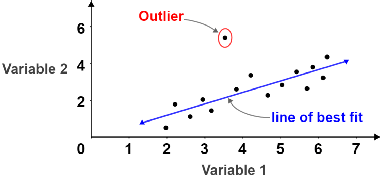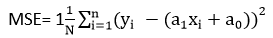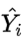# Linear Regression in ML

## Introduction

One of the simplest and most widely used Machine Learning algorithms is linear regression. The supervised machine learning model, “linear regression” finds the linear relationship between the dependent and independent variables by determining the best fit linear line between them. It is a statistical technique for performing predictive analysis. For continuous/real/numeric variables like sales, salary, age, and product price, linear regression can make predictions.

## How to find the best fit line?

Finding the best fit line, which means minimizing the difference between the predicted and actual values, is our primary objective when using linear regression. The line with the best fit will have the least error.Let us consider the above figure. For the given data points we tried to draw a line which is having the minimum error.  The line can be represented as  Y= A * X + B

where,

Y =  Dependent Variable (Actual variable )

A =  Linear regression coefficient

X = Independent Variable (predicted Variable)

B = Intercept of the line

### Cost function

We employ the Mean Squared Error (MSE) cost function for linear regression, which measures the average squared error that occurred between the predicted values and actual values.Where,

Yi = Real value

(a1xi+a0)= value which is predicted

N=Total number of observation

## Evaluation Metrics

Some statistical metrics are applied to evaluate how well your regression model is performing. Few of the metrics will be covered in this article.

### Mean Absolute Error

The absolute difference between actual and predicted values is calculated using the very simple and direct metric known as MAE.where,

N is the total number of data points

Yi is the Actual Valueis the Predicted Value

### Root Mean Squared Error

The average squared difference between the prediction and the actual value is taken to determine the Root Mean Square Error.where,

N is the total number of data points

Yi is the Actual Valueis the Predicted Value

Also read: Prediction using Linear Regression